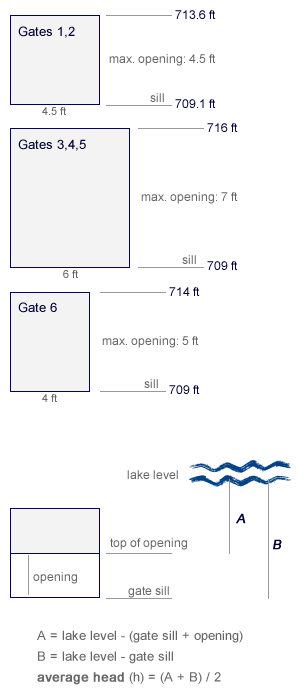# Keuka Lake Level - Calculating Total Discharge

Discharge Calculator

The six control gates for Keuka Lake are located at the Main Street bridge in Penn Yan. The three "main" gates are made of steel and have widths of 6 feet (referred to as Gates 3, 4 and 5. There are also two "Andrews" Gates that have widths of 4.5 feet (referred to as Gates 1 and 2) and the Birkett Gate (Gate 6) which is 4 feet wide.gate width gate sill max. opening Gate 1 4.5 ft 709.1 ft 4.5 ft Gate 2 4.5 ft 709.1 ft 4.5 ft Gate 3 6 ft 709 ft 7 ft Gate 4 6 ft 709 ft 7 ft Gate 5 6 ft 709 ft 7 ft Gate 6 4 ft 709 ft 5 ft

Total discharge is calculated by summing up the discharge produced
by the opening of any of these six gates. The calculation method
used are dependant on the lake elevation and, in some cases,
the gate opening.

 gate opening lake elevation method Gates 1 & 2 0.5 ft >= 709.7 ft Orifice Flow Gates 1 & 2 0.5 ft < 709.7 ft Weir Flow Gates 1 & 2 1.0 ft >= 710.3 ft Orifice Flow Gates 1 & 2 1.0 ft < 710.3 ft Weir Flow Gates 1 & 2 2 ft >= 711.5 ft Orifice Flow Gates 1 & 2 2 ft < 711.5 ft Weir Flow Gates 1 & 2 open full >= 714.5 ft Orifice Flow Gates 1 & 2 open full < 714.5 ft Weir Flow Gate 3, 4 & 5 open full >= 717.4 ft Orifice Flow Gate 3, 4 & 5 open full < 717.4ft Weir Flow Gate 6 0.417 ft >= 709.7 ft Orifice Flow Gate 6 0.417 ft < 709.7 ft Weir Flow Gate 6 0.5 ft >= 709.7 ft Orifice Flow Gate 6 0.5 ft < 709.7 ft Weir Flow Gate 6 open full >= 715 ft Orifice Flow Gate 6 open full < 715 ft Weir Flow

WEIR FLOW

Total Discharge (CFS) = discharge coefficient x gate width x (sq. root of [ lake level - sill elevation)3

• discharge coefficient = 3.16 (supplied by the U.S. Army Corps of Engineers)

ORIFICE FLOW

Total Discharge (CFS) = discharge coefficient x area x sq. root of 2gh

• discharge coefficient = 0.61 (supplied by the U.S. Army Corps of Engineers)

• Area

• top of opening = gate sill + opening

• area
• if lake level > top of opening:
• area = w x gate opening

• if lake level < top of opening:
• area = w x (lake level - gate sill)

• g = gravity = 32.15 ft/sec. squared

• if lake level > top of opening:
• h = ((lake level - gate sill) + (lake level - (gate sill + opening))) / 2

• if lake level < top of opening:
• h = lake level - gate sill

HIGH DISCHARGE FLOW EVENTS

When any gates are full open and water discharge flows are high, the impact of tailwater requires an adjustment of total flow by the Submerged Flow Correction Factor. Tailwater conditions are the result of high amounts of water on the down steam side of the gates, which then restrict the amount of water passing through the gates. The following Submerged Flow Correction Factors are used for various full gate open conditions:

 # of Gates Fully Open Submerged Flow Correction Factor When any gates are full open, the sum of the individual calculated total discharge for each open gate is then multiplied by the above appropriate Submerged Flow Correction Factor, to yield the Total Discharge for all combined gates. 1 1.00 2 1.00 3 0.99 4 0.86 5 0.75